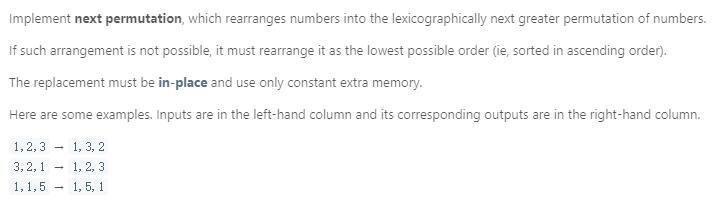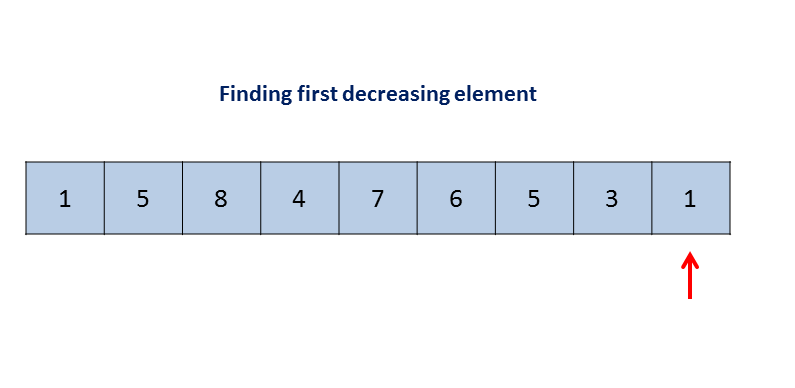# 题目描述（中等难度）# 解法一public void nextPermutation(int[] nums) {
int i = nums.length - 2;
//找到第一个不再递增的位置
while (i >= 0 && nums[i + 1] <= nums[i]) {
i--;
}
//如果到了最左边，就直接倒置输出
if (i < 0) {
reverse(nums, 0);
return;
}
//找到刚好大于 nums[i]的位置
int j = nums.length - 1;
while (j >= 0 && nums[j] <= nums[i]) {
j--;
}
//交换
swap(nums, i, j);
//利用倒置进行排序
reverse(nums, i + 1);

}

private void swap(int[] nums, int i, int j) {
int temp = nums[j];
nums[j] = nums[i];
nums[i] = temp;
}

private void reverse(int[] nums, int start) {
int i = start, j = nums.length - 1;
while (i < j) {
swap(nums, i, j);
i++;
j--;
}
}